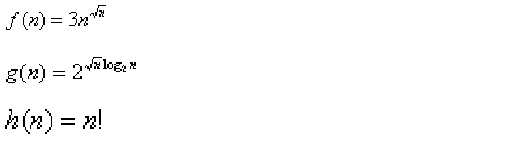# Algorithms | Analysis of Algorithms | Question 15

• Last Updated : 28 Jun, 2021

Consider the following functionsWhich of the following is true? (GATE CS 2000)
(a) h(n) is 0(f(n))
(b) h(n) is 0(g(n))
(c) g(n) is not 0(f(n))
(d) f(n) is 0(g(n))
(A) a
(B) b
(C) c
(D) d

Explanation: g(n) = 2^= n^f(n) and g(n) are of same asymptotic order and following statements are true.
f(n) = O(g(n))
g(n) = O(f(n)).

(a) and (b) are false because n! is of asymptotically higher order than n^.

Quiz of this Question

My Personal Notes arrow_drop_up